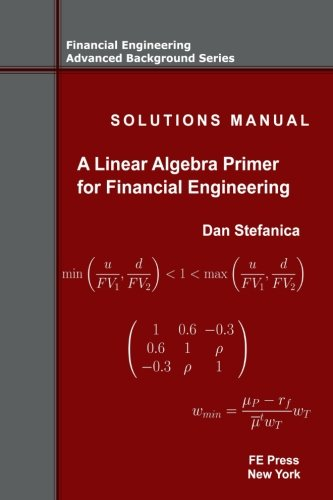# Solutions Manual – A Linear Algebra Primer for Financial Engineering (Financial Engineering Advanced Background Series) (Volume 4)Price: \$40.00
(as of Apr 03,2020 21:22:44 UTC – Details)

Every exercise from the book “A Linear Algebra Primer for Financial Engineering“ is solved in detail in the Solutions Manual.

The addition of this Solutions Manual offers the reader the opportunity of rigorous self-study of the linear algebra concepts presented in the NLA Primer, and of achieving a deeper understanding of the financial engineering applications therein.

Financial Applications

• The Arrow—Debreu one period market model

• One period index options arbitrage

• Covariance and correlation matrix estimation from time series data

• Ordinary least squares for implied volatility computation

• Minimum variance portfolios and maximum return portfolios

• Value at Risk and portfolio VaR

Linear Algebra Topics

• LU and Cholesky decompositions and linear solvers

• Optimal solvers for tridiagonal symmetric positive matrices

• Ordinary least squares and linear regression

• Linear Transformation Property

• Efficient cubic spline interpolation

• Multivariate normal random variables

Dan Stefanica has been the Director of the Baruch MFE Program since its inception in 2002, and is the author of the best-selling A Primer For The Mathematics Of Financial Engineering and A Linear Algebra Primer for Financial Engineering: Covariance Matrices, Eigenvectors, OLS, and more, and co-author of 150 Most Frequently Asked Questions on Quant Interviews. He teaches graduate courses on numerical methods for financial engineering, as well as pre-program courses on advanced calculus and numerical linear algebra with financial applications. His research spans numerical analysis, graph theory, and geophysical fluid dynamics. He has a PhD in mathematics from New York University and taught previously at the Massachusetts Institute of Technology.